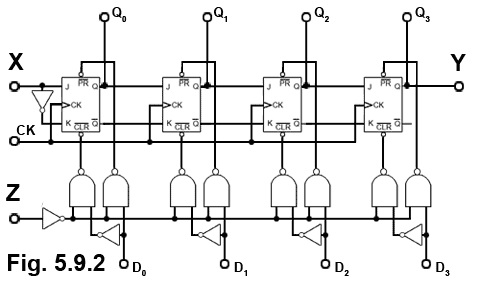# Sequential Logic Quiz

Try our quiz, based on the information you can find in Digital Electronics Module 5 - Sequential Logic. Submit your answers and see how many you get right. If you get any answers wrong. Just follow the hints to find the right answer and learn more about sequential logic circuits as you go.

### 1.

Which of the listed flip-flops is also known as a programmable flip-flop?

### 2.

Which of the following is a major advantage of the D-type flip-flop over the clocked SR type?

### 3.

Which of the following is a major advantage of synchronous counters over asynchronous counters?

### 4.

Which of the following actions must an ALU perform to Negate the input data?

### 5.

In a simple low activated SR flip-flop, the Q and NOT Q will be indeterminate (unknown) if:

### 6.What type of device is shown in Fig. 5.9.1 if the waveforms illustrated are present at its CK and Q terminals?

### 7.What type of circuit is illustrated in Fig. 5.9.2?

### 8.What type of circuit is illustrated by Fig. 5.9.3?

### 9.

Refer to Fig. 5.9.3. What is the function of the input marked X?

### 10.Refer to Fig. 5.9.4. After 2 more clock pulses what will be the logic states on outputs A, B, C, D and E?

Top of Page